# Dot Product 101

### Why should I care about the Dot Product?

Vectors are very common in game development.  We use them for all sorts of things like determining where the player is facing, the speed and direction of projectiles, how much light a polygon should receive, and even defining the polygon itself!  The dot product is the foundation of all matrix multiplication.  Infact, as we will soon see, the dot product is the foundation for many other advanced vector manipulation mathematics.  So a good insight into what the dot product is and how it can be used will prove to be very useful in game development.

### What does the Dot Product mean?

Due to the frequent use of vectors in a game engine, often times we need to understand relationships between vectors.  The dot product allows us to see how similar 2 vectors are. When 2 vectors are normalized, the dot product of both vectors is the length of one vector projected onto the other.  The more positive the dot product, the more similar the vectors are.  The more negative the dot product, the more opposite the vectors are.  Take a look at Figure 1 to get a geometric interpretation of the dot product.

Figure 1.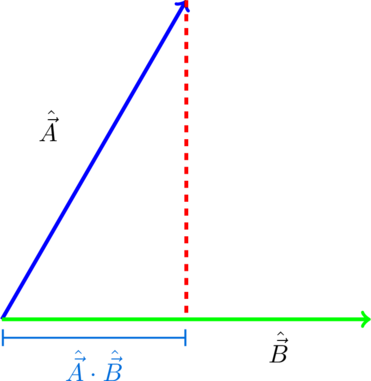In figure 1, vectors A and B are both normalized vectors meaning each of their lengths are equal to 1. When you take the dot product of two normalized vectors, the result will be the length of the first vector projected onto the second vector.

### How do I calculate the Dot Product?

The Dot product is pretty straight forward to calculate.  It also has some interesting properties.  Figure 2 is the classic dot product formula.

Figure 2.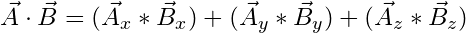Whereas Figure 3 is more of a trigonometric perspective of the Dot Product.

Figure 3.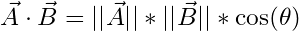### How can I use the Dot Product algebraically?

The following are some of the algebraic properties of the Dot Product.

Commutative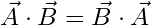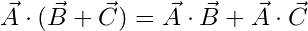### What kinds of questions can I ask about vectors using the Dot Product?

We can ask all kinds of questions about Vectors using the Dot product!

##### What is the angle between two vectors?

We can exploit the equation listed in Figure 3 like so to derive the angle.

Figure 4.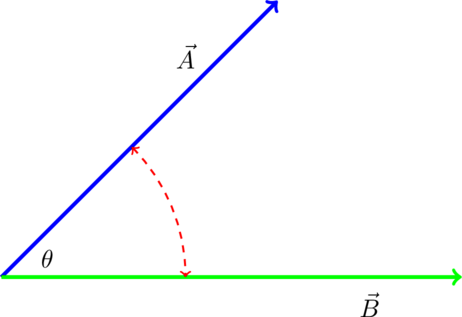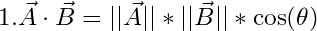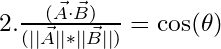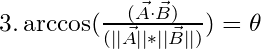##### Are 2 vectors acute, perpendicular, or obtuse?

Back in the day, using trig functions like sin,cosine,tangent, etc were quite expensive.  They were so expensive that many developers used look up tables to increase performance.  However as CPUs continued to become more performant, using trig functions became less of an issue.  Even so, they still are not the cheapest operation on the block and if you just want to know whether or not 2 vectors are perpendicular/acute/ or obtuse, there are far cheaper ways of figuring that out than using a trig function.  After all, why waste precious CPU cycles when you can spend them on better graphics?

Figure 5.
Perpendicular Vectors have a dot product equal to 0.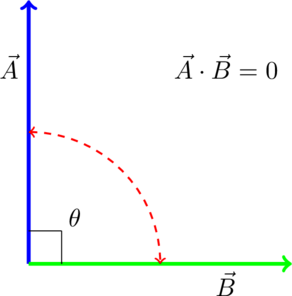Acute Vectors have a dot product greater than 0.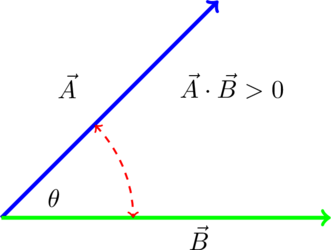Obtuse Vectors have a dot product less than 0.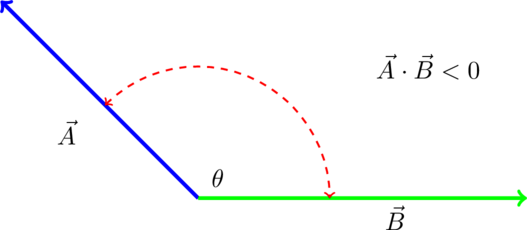### What are some practical use cases of the Dot Product?

##### Determining if the player is in front or behind an enemy.

If you are making an FPS or stealth game, you will probably only want the enemy to engage the player if he sees the player which means the player should be in front of the enemy. You can easily accomplish this using the dot product.

Figure 6.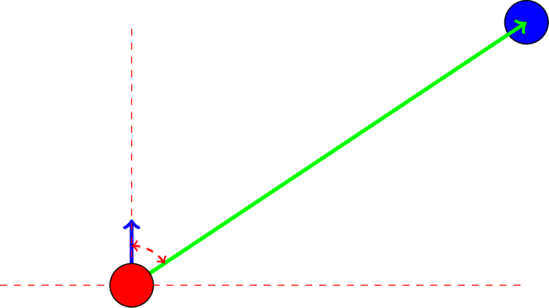In this case, you need to take the dot product between the Enemy’s look vector and the vector from the Enemy to the Player. If the dot product result is greater than 0, then the player is in front of the Enemy. If the result if less than 0, the player is behind the enemy.

##### Project a vector onto another vector

If you know that the dot product is just the length of one vector projected onto another, you can easily derive the formula for getting the actual projected vector without having to look up the formula in a textbook. Take another look at the following visualization of the dot product.

Figure 7.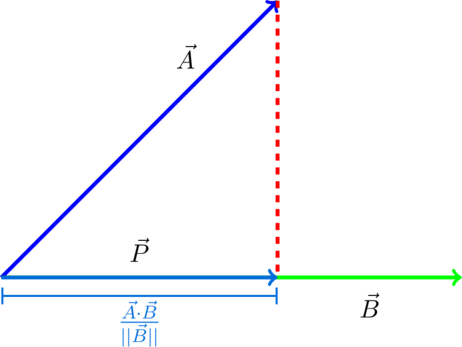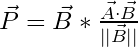Or if both A and B are normalized, then you can get the projected point P like so,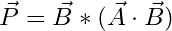###### Code Sample:

The reason why we have to divide the dot product by the magnitude or length of B in the non-normalized case is because we want to create a scalar, preferably between 0 and 1, by which we can scale the vector B by. Since the dot product of A and B is within the same length space of vector B, we can just divide that length by the total length of B to determine what percentage of vector B is made up by our resulting vector P. In other words, we need to know how much of vector B is vector P so that we can scale vector B by an amount that transforms B into P.

##### Reflecting a Vector

Imagine you were making a breakout or pong game. What would you do once the ball collides with the paddle? Typically, the ball when bounce off the paddle in the opposite direction from which it came. So you would want to essentially reflect the incoming vector V about the paddle’s normal vector N to get the reflected vector R as shown in figure 8.

Figure 8.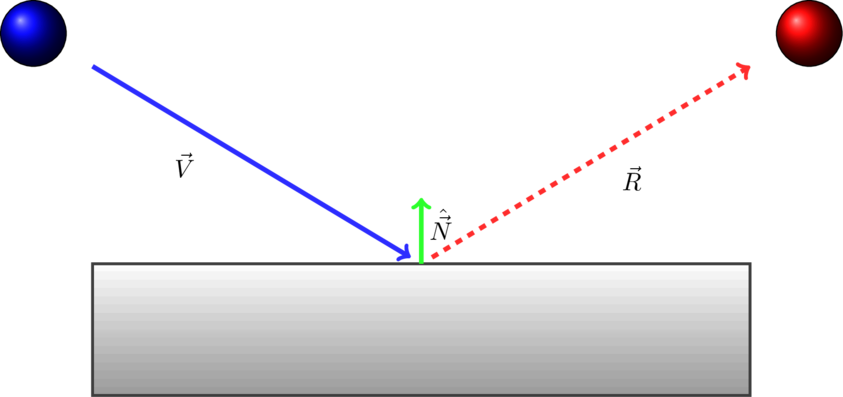Our end goal is to find the reflected vector R.The only givens are vectors V and N. To make it harder, neither vector is normalized.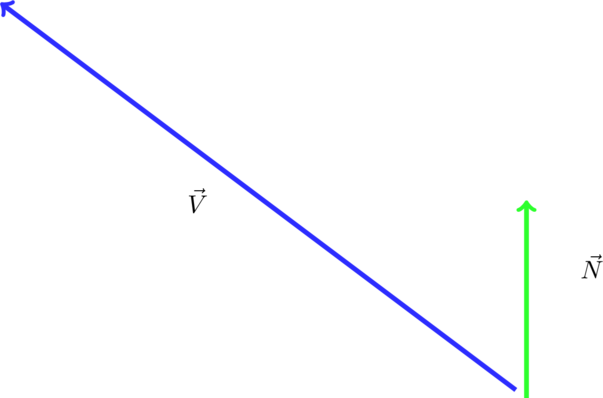So first, lets project V onto N to get V Prime.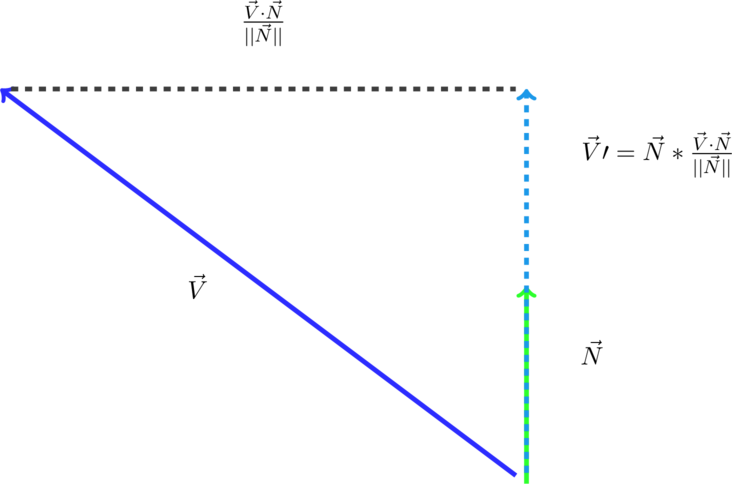Now we know that V prime, the projected Vector V that is, is the same height as vector V. So lets double the length of V prime.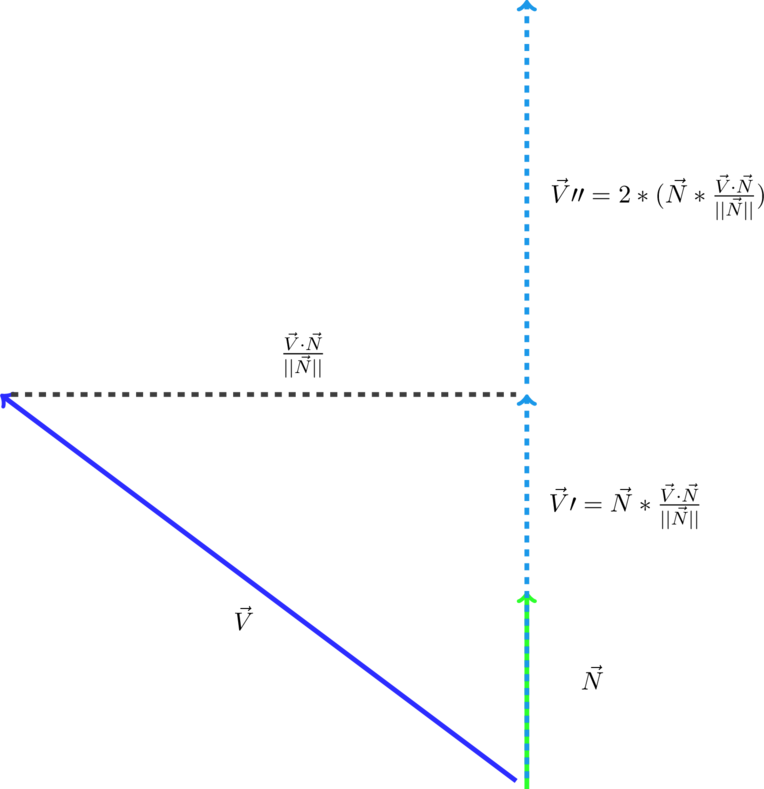Our reflection vector can now be computed as the vector from V to V Prime Prime. The reason this works is because if our projected vector, V Prime, is at the same height as our vector V, then doubling the projected vector’s length will make our original vector travel just as far as our reflected vector would. All we really need to do is mentally translate this vector back to the origin.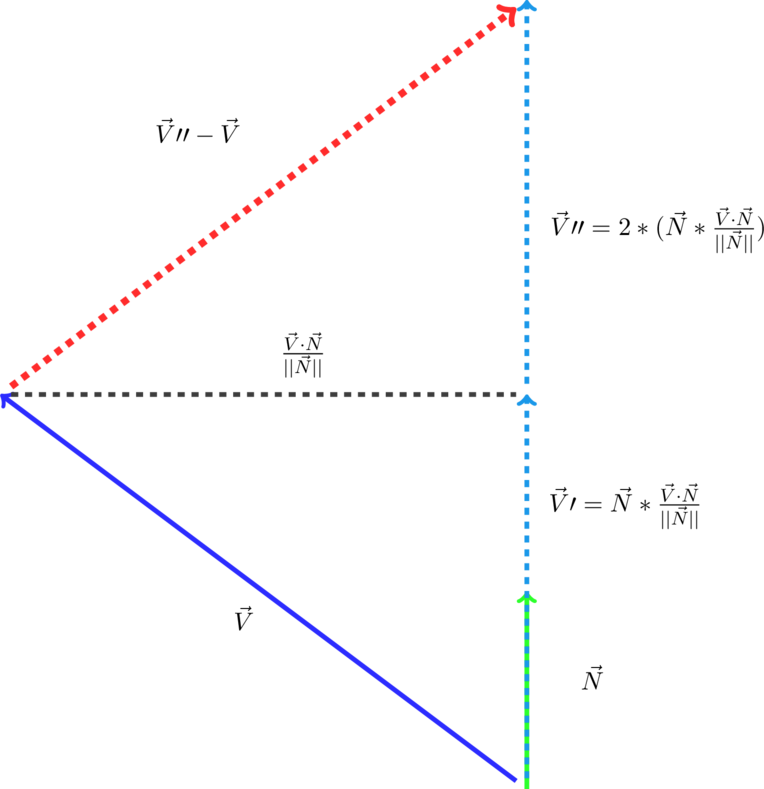Finally we have our reflected vector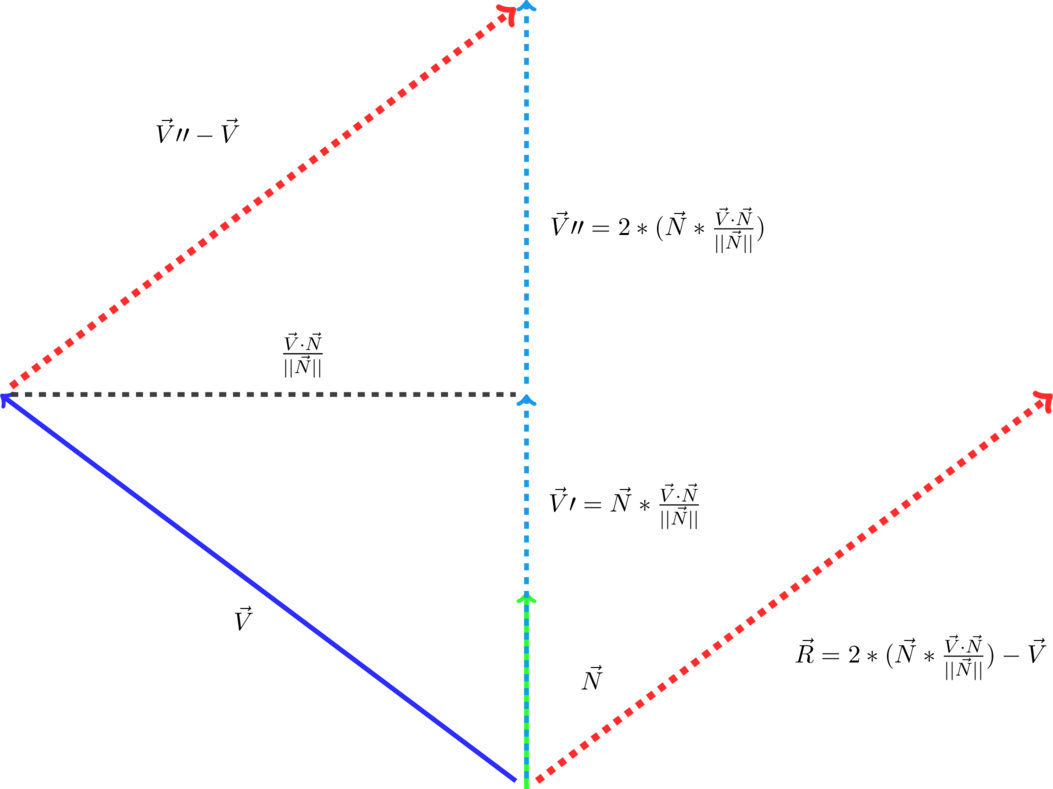Therefore the formula for reflecting a vector is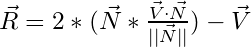##### Calculate the Length of a Vector Efficiently

The formula for calculating the length of a 3d vector is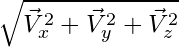###### Code Sample:

The problem is that the square root function is generally not very cheap to calculate and should be avoided when possible. Sometimes when you need the length of a vector, you may actually be able to get away with just using the squared length which is much cheaper to calculate. For example, in the following code sample, I needed to know how far the player is from the enemy. If the enemy is within 4 units of the player, then I want the enemy to attack the player.

If we just want the squared length of a vector, we can just take the dot product of that single vector.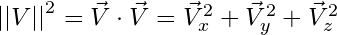##### Matrix Multiplication

Matrix multiplication is a core mathematical operation that takes place all the time in 3D video games. Every time your character rotates or changes his position, there are many matrix multiplications happening behind the scene to cause those various kinds of transformations.

But just what is Matrix Multiplication? It is just a series of dot products between the rows in one matrix and the columns in another matrix.

Figure 9.### Conclusion

By now, if I have done my job well, you should be convinced that the dot product is a very powerful and common function that is used in game development. The dot product allows us to ask questions regarding how similar 2 vectors are. Core game systems like graphics, ai, gameplay, physics, and sound have to solve 2d and 3d problems quite frequently. Therefore the dot product is used very often to determine relationships between various vectors. I hope you learned something from this article. Stay tuned for more math and graphics related articles on my article page.